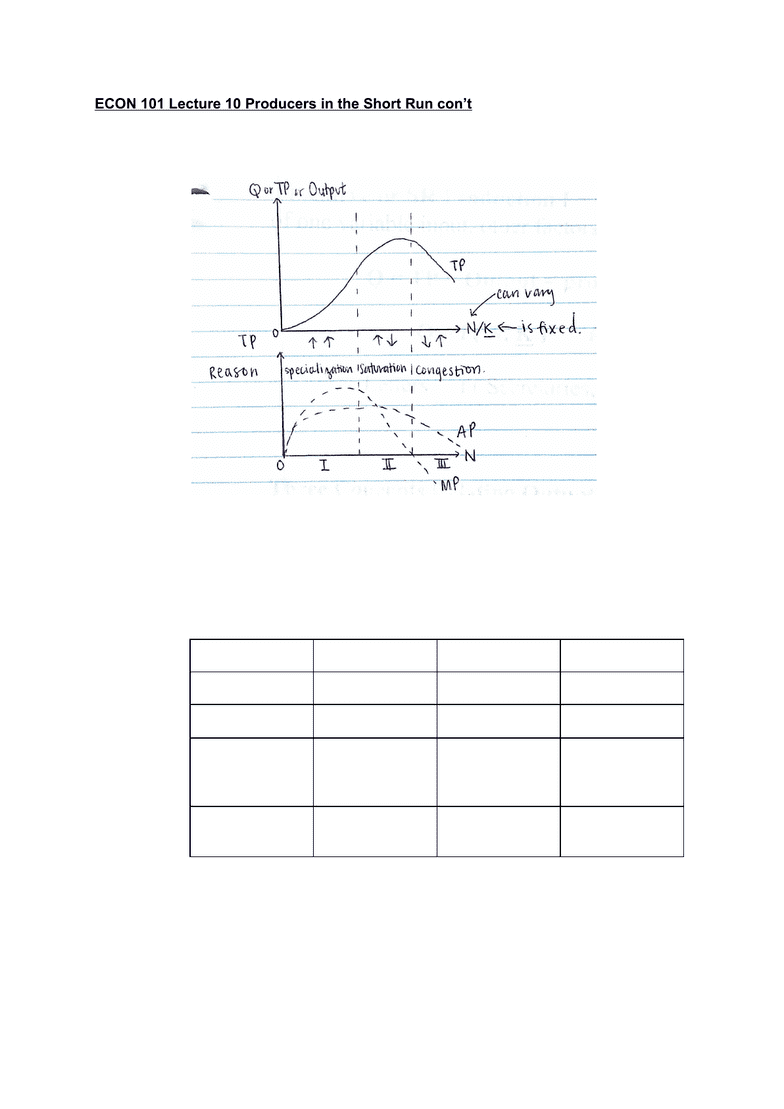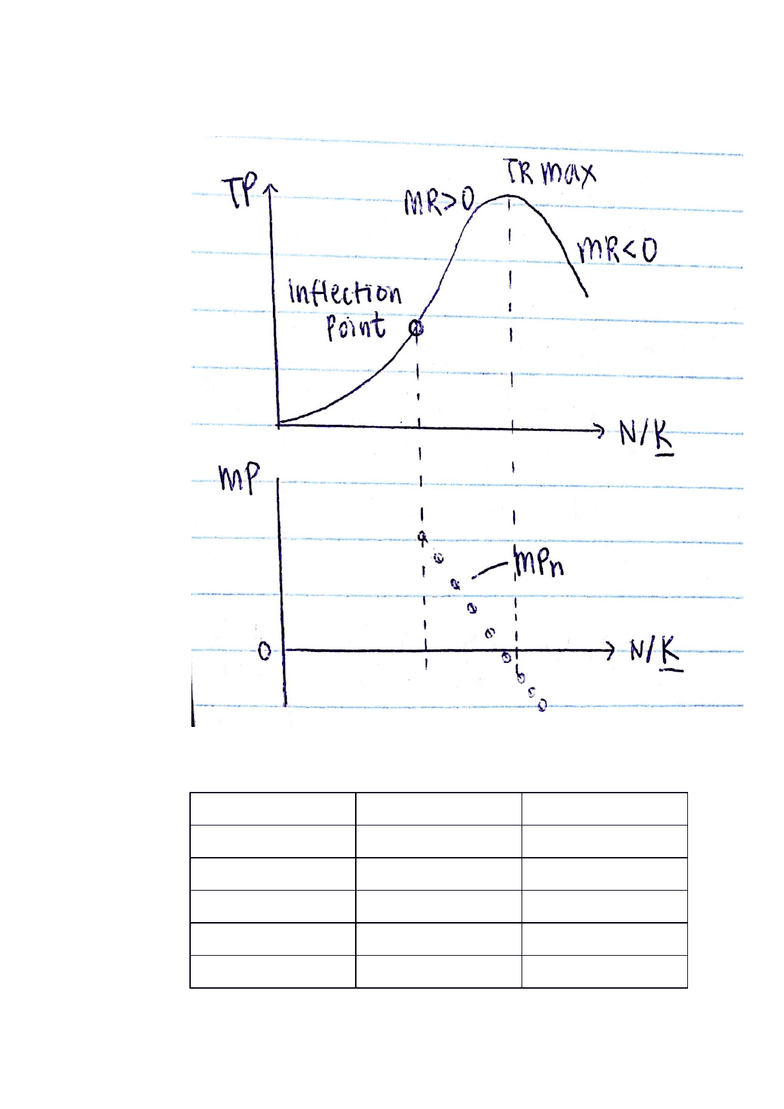Class Notes (1,100,000)
CA (650,000)
UBC (10,000)
ECON (800)
ECON 101 (200)
Lecture 10

# ECON 101 Lecture Notes - Lecture 10: Ceteris Paribus, Inflection Point, Marginal ProductPremium

Department
Economics
Course Code
ECON 101
Professor
Robert Gateman
Lecture
10

This preview shows page 1. to view the full 5 pages of the document.ECON 101 Lecture 10 Producers in the Short Run cont
1. Production in the short run
a. Total, average, marginal product SR Production Function
b.
c. TP Curve or SR Production Fxn
i. measures output as a function of one variable input, other factors held
constant (partial derivatives)
ii. Q = TP = Output = production
iii. E.g. Q = f(N, K) (labour varies, K is constant)
d. Three concepts relating output Q to variable Factor N
i.
Total Product
Average Product
Marginal Product
Symbol
TP or Q
AP
MP
Definition
production
AP = TP/N
MP = ΔTP/ΔN
Explanation
production
Total output per
unit of variable
factor, N
Extra output
form one last
unit of input
Graphically
Lazy “S”
Slope of ray
from origin
Slope of tangent
ii. TP curve used to derive TC curve
e. Law of diminishing marginal product (returns)
f. LDMP or LDMReturns (don’t confuse with MREvenue)
i. Is the idea that as more of the variable factor is added to a given
amount of the fixed factor, the additional output diminishes after a
certain point (Inflection point), ceteris paribus
ii. Reason:

Unlock to view full version

Subscribers Only

Only page 1 are available for preview. Some parts have been intentionally blurred.

Subscribers Only1. More variable factor has less of the fixed factor to work with!
2. Same reason as the shape of the mother of all curves, the TP
g.
2. Cost in the short run
a. Total, average, marginal costs
i.
Type of cost
Symbol
Definition
Total costs
Total Fixed Costs
TFC
Does not ~ Q
Total Variable
TVC
Does ~ Q
Total Costs
TC
Pf x Qf
Average Costs